Next: Worked example 5.3: Stretching Up: Conservation of energy Previous: Worked example 5.1: Bucket

## Worked example 5.2: Dragging a treasure chest

Question: A pirate drags a 50kg treasure chest over the rough surface of a dock by exerting a constant force of 95N acting at an angle of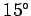above the horizontal. The chest moves 6m in a straight line, and the coefficient of kinetic friction between the chest and the dock is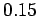. How much work does the pirate perform? How much energy is dissipated as heat via friction? What is the final velocity of the chest?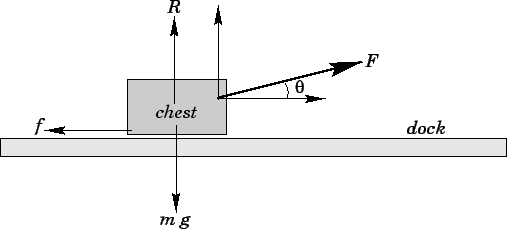Answer: Referring to the diagram, the forceexerted by the pirate can be resolved into a horizontal component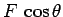and a vertical component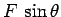. Since the chest only moves horizontally, the vertical component ofperforms zero work. The work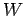performed by the horizontal component is simply the magnitude of this component times the horizontal distancemoved by the chest: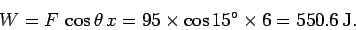The chest is subject to the following forces in the vertical direction: the downward force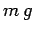due to gravity, the upward reaction forcedue to the dock, and the upward componentof the force exerted by the pirate. Since the chest does not accelerate in the vertical direction, these forces must balance. Hence,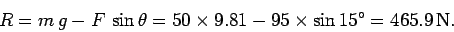The frictional forceis the product of the coefficient of kinetic frictionand the normal reaction, so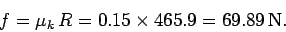The work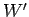done by the frictional force is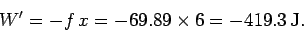Note that there is a minus sign in front of thebecause the displacement of the chest is in the opposite direction to the frictional force. The fact thatis negative indicates a loss of energy by the chest: this energy is dissipated as heat via friction. Hence, the dissipated energy is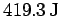.

The final kinetic energyof the chest (assuming that it is initially at rest) is the difference between the workdone by the pirate and the energy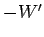dissipated as heat. Hence,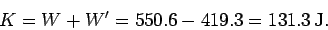Since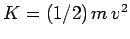, the final velocity of the chest is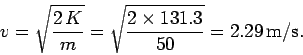Next: Worked example 5.3: Stretching Up: Conservation of energy Previous: Worked example 5.1: Bucket
Richard Fitzpatrick 2006-02-02